﻿ Starting Characteristics of Induction MotorExamples | Product | Murata Software Co., Ltd.Example4Starting Characteristics of Induction Motor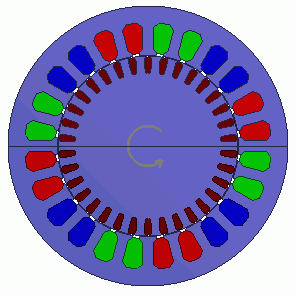General

• A cage structure three-phase induction motor is analyzed.
The magnetic field of coils on the stator generates eddy current at the conductor on the rotor.
The magnetic fields generated by eddy current and stator create the torque which rotates the asynchronous motor.

• The motion coupled analysis is applied.

• The analysis begins from the stationary state of the model. The rotation is gradually increased and reaches the stable state at about 1000[r/min].

• The rotation velocity, torque and magnetic flux density are solved.

• Unless specified in the list below, the default conditions will be applied.

Analysis Conditions

 Item Setting Solver Magnetic Field Analysis [Luvens] Analysis Space 2D Analysis Type Transient Analysis Unit mm Options Select External Circuit Coupling. Select Rotating machinery.   [Conversion] Model Thickness: 4.2×10^-2[m] Select Partial Model. Number of Divisions of the Whole Model: 2 Circuit Configuration Number of Series: 1 Circuit Configuration Number of parallels: 2 Select Convert the result to the whole model and output it.

The Rotating Machinery tab is set as follows.

 Tab Setting Item Setting Rotating Machinery Rotational Movement Select Motion Equation Coupling Number of Slide Mesh Divisions Circumferential Division Angle: 0.5[deg] Number of Slide Mesh Layers: 3 Setting Motion Equation Coupling Moment of Inertia Inertial Moment of Rotor: 6.269×10^-4[kg*m2] Inertial Moment of Load: 3.0×10^-3[kg*m2] External Torque Waveform: Constant 0[N*m] Coulomb Friction Torque Waveform: Coefficient Coulomb Friction Torque: 0.5×10^-5[N*m] Viscous Friction Coefficient: 0[N*m/(r/min)] Squared Load Torque Coefficient: 1.226×10^-6[N・m/(r/min)2] Initial Time State Number of Rotations: 0[r/min] Rotor’s Initial Rotation Position: 0[deg]

External circuit is as follows.

50[Hz] three-phase AC voltage is applied.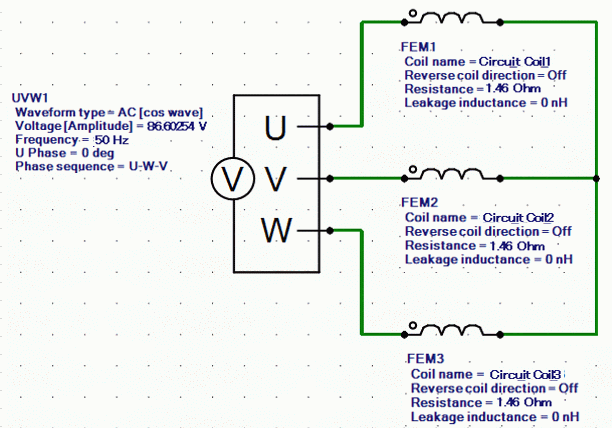Set the Mesh Tab as follows.

 Tab Setting Item Setting Mesh Meshing Setup Automatically set the general mesh size: Deselect General mesh size: 2[mm] Ambient Air Creation Select Create ambient air automatically Ambient Air Scale: 1.2

The Transient Analysis tab is set up as follows.

One second is divided into 800 steps.

Tab

Setting Item

Setting

Transient Analysis

Time step

Manual

Table

 Number Calculation steps Output steps Time step [s] 1 800 1 1.0/800

Graphical Objects

A rotor cage consisted of conductor and rotor core is placed in the center. A stator core and coils are placed around it.

This is a 2D model analysis. By utilizing the symmetry of the analysis model, it is made to be a half period symmetric model.

“Ambient Air Creation” is selected.

Small mesh size is (0.3mm) is applied to the gap.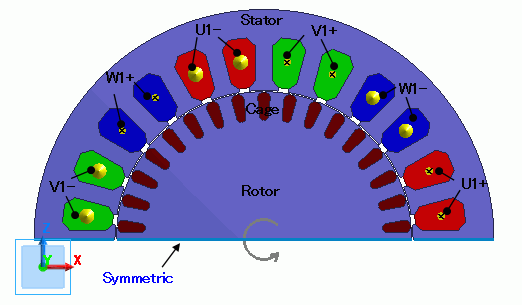Body Attributes and Materials

 Body Number/Type Body Attribute Name Material Name 25/Sheet Cage Al 26/Sheet Cage Al 27/Sheet Cage Al 28/Sheet Cage Al 29/Sheet Cage Al 30/Sheet Cage Al 31/Sheet Cage Al 32/Sheet Cage Al 33/Sheet Cage Al 34/Sheet Cage Al 35/Sheet Cage Al 36/Sheet Cage Al 37/Sheet Cage Al 38/Sheet Cage Al 39/Sheet Cage Al 40/Sheet Cage Al 41/Sheet Cage Al 24/Sheet Rotor 50A1300 65/Sheet Stator 50A1300 67/Sheet U1+ Cu 68/Sheet U1+ Cu 69/Sheet W1- Cu 70/Sheet W1- Cu 71/Sheet V1+ Cu 72/Sheet V1+ Cu 73/Sheet U1- Cu 74/Sheet U1- Cu 75/Sheet W1+ Cu 76/Sheet W1+ Cu 77/Sheet V1- Cu 78/Sheet V1- Cu

The material properties are set up as follows:

 Body Attribute Name Tab Settings Cage Stator/Rotor/Air Rotor Rotor Stator/Rotor/Air Rotor Stator Stator/Rotor/Air Stator U1- Current Waveform: External Circuit Coupling Coil name on the circuit: U1 Turns: 66[Turns] Direction: – Y Direction Stator/Rotor/Air Stator U1+ Current Waveform: External Circuit Coupling Coil name on the circuit: U1 Turns: 66[Turns] Direction: +Y Direction Stator/Rotor/Air Stator V1- Current Waveform: External Circuit Coupling Coil name on the circuit: V1 Turns: 66[Turns] Direction: – Y Direction Stator/Rotor/Air Stator V1+ Current Waveform: External Circuit Coupling Coil name on the circuit: V1 Turns: 66[Turns] Direction: +Y Direction Stator/Rotor/Air Stator W1- Current Waveform: External Circuit Coupling Coil name on the circuit: W1 Turns: 66[Turns] Direction: – Y Direction Stator/Rotor/Air Stator W1+ Current Waveform: External Circuit Coupling Coil name on the circuit: W1 Turns: 66[Turns] Direction: +Y Direction Stator/Rotor/Air Stator

The material properties are set as follows.

Material Name

Tab

Properties

Al

Electric Conductivity

Conductivity Type: Conductor

Conductivity: 1.02×10^7[S/m]

Cu

Electric Conductivity

Conductivity Type: Conductor

Conductivity: 5.977×10^7[S/m]

50A1300

Permeability

Magnetization Characteristic Type: Select B-H curve

B-H Curve Table

 Magnetic Field [A/m] Magnetic Flux Density [T] 0 0 20 0.028 30 0.035 35 0.038 40 0.04 45 0.042 50 0.044 60 0.058 70 0.07 80 0.085 90 0.11 100 0.135 120 0.215 140 0.335 160 0.48 200 0.86 240 1.12 300 1.29 400 1.431 500 1.491 600 1.531 700 1.559 800 1.576 900 1.581 1000 1.591 1300 1.617 1600 1.63 2200 1.658 3000 1.689 4000 1.725 5000 1.756 7000 1.819 10000 1.888 16000 1.975 22000 2.026 30000 2.078 40000 2.122 50000 2.163 70000 2.238 80000 2.271

Boundary Conditions

Symmetric period boundary is set.

 Boundary Condition Name/Topology Tab Boundary Condition Type Setting Symmetric Symmetry/Continuity Periodic Rotation Period (1 period)

Results

The distribution of the magnetic flux density right after the start is shown below.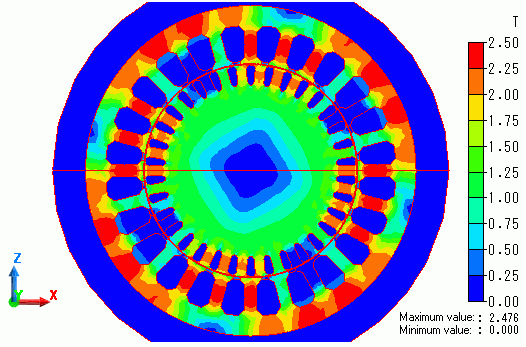The distribution of eddy current density is shown as follows.

Eddy current is generated at the cage conductor by the magnetic flux created by the coils.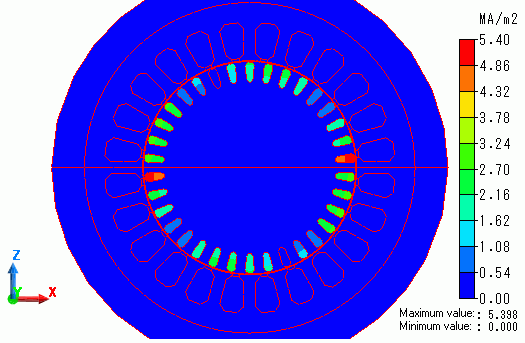The diagram below shows time-rotation velocity characteristic.

The rotation is gradually increased and reaches the stable state at about 1000[r/min].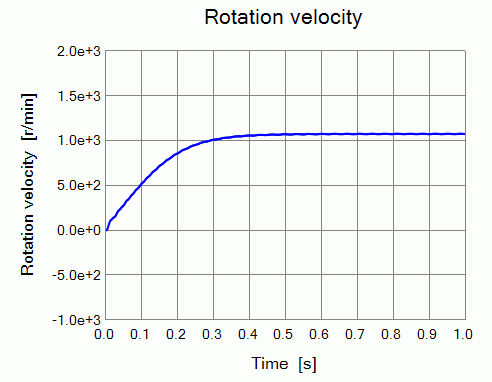The diagram below shows time-torque characteristics.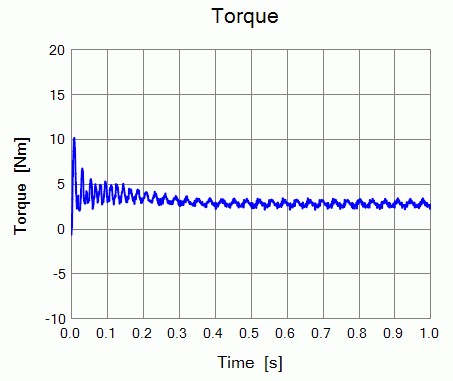﻿